Blogg | Combine

BloggOr ”can daedalean words actually help make more accurate descriptions of your random variable? Part 1: Kurtosis

Is a common belief that gaussians and uniform distributions will take you a long way.
Which is understandable if one considers the law of large numbers: with a large enough number of trials, the mean converges to the expectation. Depending on your reality, you may not have a large enough number of trials. You could always calculate an expected value out of that (limited) number of trials, and for certain conditions on your random variable, you could get a bound on the variation of that expectation, together with a probability of that bound. And that alone is a lot of information on how your random variable might behave.

But you could do a bit more with those expected value calculations! Especially if you have a reason to believe that the best fit for your random variable might not be normal, yet you simply don’t have enough samples to commit to a model right now. Could it be that the apparent misbehavior of a random variable is actually a feature instead of a bug?

Kurtosis: Peakyness, Broad-shoulderness and Heavy-tailedness

Kurtosis (we can use κ interchangeably to denote it) describes both the peakyness and the tailedness in a distribution. κ can be expressed in terms of the expectation:

β_2 = E(X-μ)^4 / (E(X-μ)^2)^2

Or in terms of the sample means (a.k.a. sample κ):

b_2 = (Sum((Xi- μ{hat})^4)/n) / (Sum((Xi-μ{hat})^2)/n)^2

Kurtosis represents a movement of mass that does not affect the variance and reflects the shape of a distribution apart from its variance. Is defined in terms of an even power of the standard score, so is invariant under linear transformations. Normal distributions have the nice property of having κ = 3. Take the following re-enactment of the wikipedia illustration for normal distributions: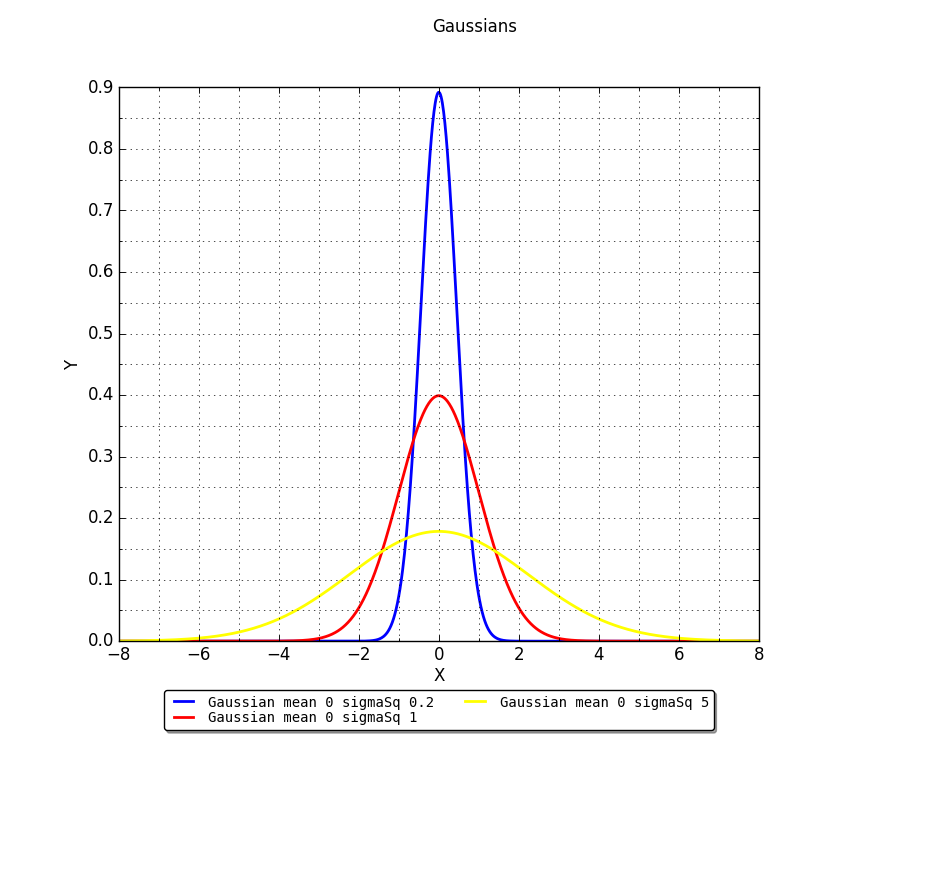As you may have guessed from the labels, we have plotted three gaussians with different σ^2 (0.2, 1, 5), all with mean zero. If we draw samples from these distributions and apply the sample κ expressions, we will in all cases get κ ~3. All these three distributions have the same Kurtosis. You can try with sample sizes as small as 9, and move all the way to 1000, 3000 and more. You will still get κ ~3. This is why there is a relative measure called Excess Kurtosis, which is the kurtosis of a distribution with respect that of a normal distribution. And as you may have guessed, is built with  κ – 3.

Distributions with negative  κ – 3 are known as platykurtic. The term ”Platykurtosis” refers to the case in which a distribution is Platykurtic. Relative to the normal, platykurtosis occurs with light tails, relatively flatter center and heavier shoulders.

Distributions with excess kurtosis (with a positive κ – 3) are known as leptokurtic. Just as with Platykurtosis, the term ”Leptokurtosis” refers to the case in which a distribution is Leptokurtic. When Leptokurtosis occurrs, heavier tails are often accompanied by a higher peak. Is easier to think of hungry tails eating variance from the shoulders/tips or ”thinning” them (the greek word ”Lepto” means ”thin, slender”).

So excess kurtosis on most cases captures how much of the variance would go from the shoulders to the tails and to grow the peak (leptokurtosis) or from the tails and the peak height into the shoulders (platykurtosis). Leptokurtosis can either occur because the underlying distribution is not normal, or because outliers are present. So if you are sure that your underlying phenomenon is normal yet you experience leptokurtosis, then you can either re-evaluate your assumptions, or consider the presence of outliers.

Detecting Bimodality

According to De Carlo (first reference of this post) Finucan in his 1964 ”A note on Kurtosis” noted that, since bimodal distributions can be viewed as having heavy shoulders, they should tend to have negative kurtosis. Moreover, Darlington in ”Is kurtosis really ’peakedness’?” (1970) argued that excess kurtosis can be interpreted as a measure of unimodality versus bimodality, in which with large negative kurtosis is related to bimodality, with the uniform distribution (κ = -1.2) setting a dividing point.

Discussing Darlington’s results and Finucan’s note would probably require another post… Let’s see how we go for that later :). For now, I think the following plot from wikipedia shows all the former behaviours really nicely: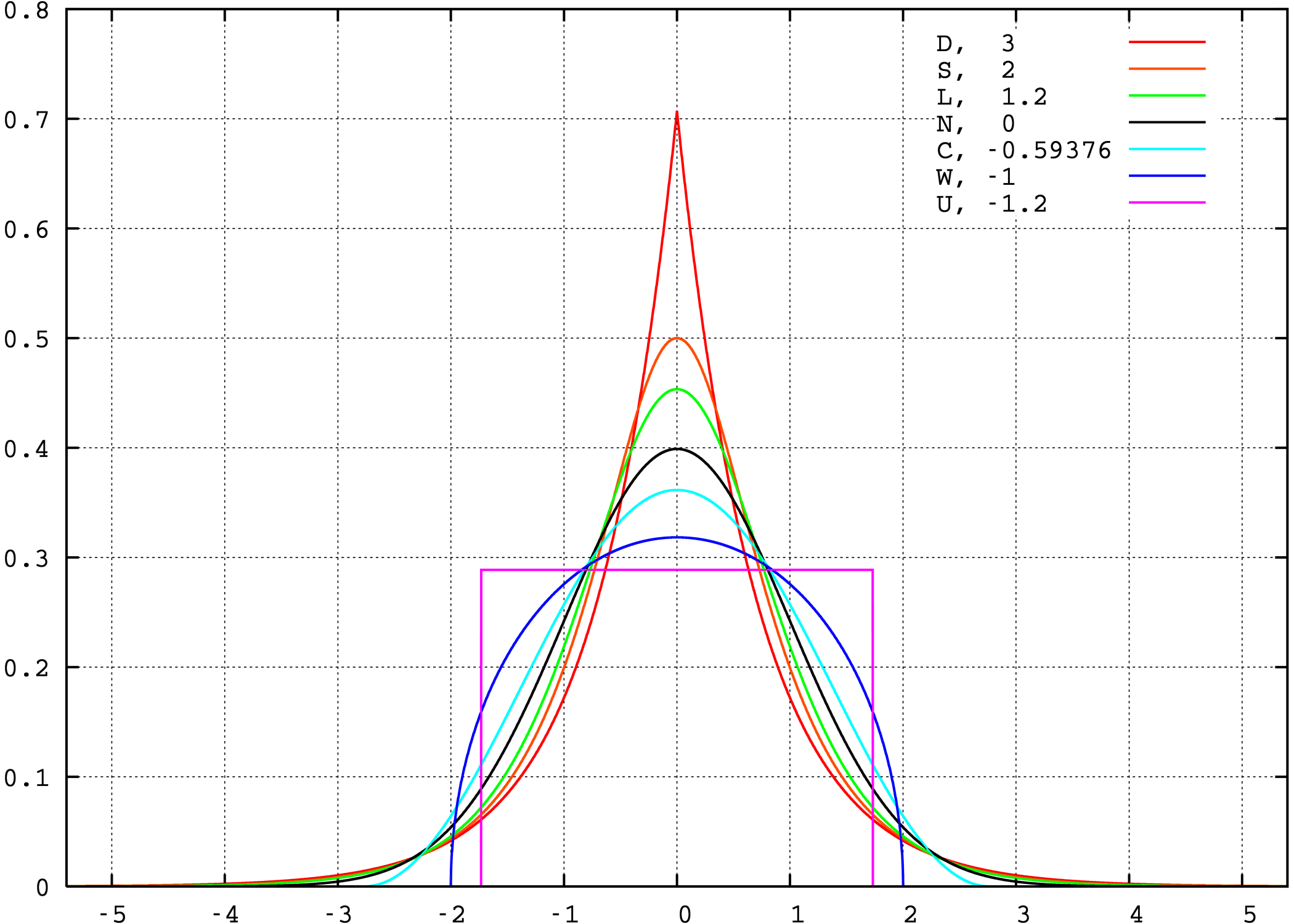Kurtosis for assessing normality

Gaussians are like sedans: you can drive them in the city, you can drive them in the highway, you can drive them to the country. They will take you thru many roads. But sometimes you need a proper truck. Or fabulous roller-skates. And knowing when would you need either can save you from toiling.

Thanks to the higher moments of a distribution, is possible to make relatively cheap tests for normality, even with really small sample sizes. If you wanted to compare the shape of a (univariate) distribution relative to the normal distribution, you can by all means use Excess Kurtosis. Just calculate sample kurtosis for the data you are studying. If it deviates significantly from 3, even for a small number of samples, then you are either not dealing with a gaussian distribution, or there is too much noise for such a sample size.

Multivariate normality & multivariate methods

The assumption of normality in the multivariate case prevails in many application fields, often without verifying how reasonable it would be in each particular case. There is a number of other conditions to check for multivariate normality. A property of multivariate normality is that all the marginals are also normal, so this should first be checked (this can be quickly performed with excess kurtosis). Also, linear combinations of the marginals should also be normal, squared Mahalanobis distances have an approximate chi-squared distribution (often q-q plots are used for this purpose), and we can go on. You could even use this web interface to an R package dedicated exclusively to check multivariate normality.

Kurtosis can affect your study more than you think. When applying a method for studying your random variable, keep in mind that some methods can be more or less affected by the higher moments of the distribution. Some methods are better at dealing with some skewdness (for another post) while some tests are more affected by it. Similarly with Kurtosis. Tests of equality of covariance matrices are known to be affected by kurtosis. Analyses based on covariance matrices (shout out PC Analysis!) can be affected by kurtosis. Kurtosis can affect significance tests and standard errors of parameter estimates.

Final words

The contents of this post have been influenced by:

Is too bad that I could not find a way to read or buy Finucan’s note on kurtosis, because it seemed interesting. If anyone has access to it, please comment and let me know how to get it.

There is more than Monte Carlo when talking about randomized algorithms. It is not uncommon to see the expresions ”Monte Carlo Approach” and ”randomized approach” used interchangeably. More than once you start reading a paper or listening to a presentation, in which the words ”Monte Carlo” appear on the keywords and even on the title, and as you keep reading/listening, you notice in the algorithm description a part in which the runs with incorrect outputs are discarded until only correct outputs are given… Which effectively turns a Monte Carlo into a Las Vegas. Running time is no longer deterministic-ish, and the algorithm will only provide correct answers. So, they say/write ”Monte Carlo” here, ”Monte Carlo” there, and when it comes to what actually happens, you are not really in Monte Carlo, and you might be in Vegas, baby.

You can do a small check yourself. Use your favorite search engine with ”monte carlo simulation” and ”las vegas simulation”. Depending on your search engine, you may get something looking more or less like this:
aprox 84 200 000 results (0,32 seconds) – monte carlo simulation

aprox   9 560 000 results (0,56 seconds) – las vegas simulation

Almost a whole order of magnitude more in relevant results from Monte Carlo, in almost half the time. Now, if you used something like google, you may or may not get relevant results in your first screen, depending on how well google knows you. Of course, there are the cases of ”monte carlo simulation” that most likely refer to other topics e.g. Monte Carlo integration and the like, the same with ”las vegas simulation”. And depending on how much your search engine knows your habits, you might not get any results of Las Vegas simulations right away.

And probably the next thing you may do, is to do a quick wikipedia search of ”Las Vegas algorithm”. You may possibly read it, and find in the end ”Atlantic City algorithm”. And maybe you may want to follow that little wikipedia rabbit hole, and end up reading about it. And then no one can save you.

The featured image in this post is from here.

Remember your friend from our very first post? . Well, I am sorry to say that he never really reached French Guyana. He ended up in Carcass, one of the Malvinas/Falkland islands. And his boat was (peacefully) captured by overly friendly pirate penguins. Now he spends his days counting penguins and sheep. He did keep a coin and this calculator as a memento from his past life. You know, just in case.

As for you, you are still at the office and now your task is to get some samples from a distribution that strangely resembles a cropped half co-sine. Your problem is that such distribution is not in NumPy (gasp!). Don’t fret, we got you covered. NumPy not having a distribution should not cause weeping or gnashing of teeth. In fact, your friend can easily build pretty much any distribution with the help of some math, his coin and his trusty office memento (provided that the coin is unbiased and that he has enough patience). This is possible because of the Probability Integral Transform (PIT). As part of this tutorial, we will do the exercise in slide 11 of this lecture, so you might want to open the slides to do the steps together.

One consequence of the PIT is that as long as you can have a random variable in which a cumulative distribution function (CDF) exists, you can then transform the CDF to express the random variable in terms of the uniform distribution. Which means that for a very long list of distributions it would be possible for you to build your own random number generator, using nothing but some simple functions and a uniform distribution sampler!

So, let’s begin with the exercise of the tutorial. We will use our trusty calculator node, so you can place 3 calculator nodes and a dummy input (which can easily be built with a random table generator). We will not use the actual contents of the dummy input, since we will generate our own signals in the calculator. Your start flow may look like this: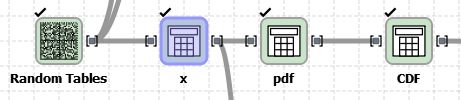You can change the labels in the node by double clicking the text. Now, right click on the first calculator node (from left to right) and click ”configure”. Make a variable that will be your independent axis through the calculations. If you look in the exercise, the range of the pdf goes from (-pi/2 to pi/2]. Note that the lower bound is an open bound. I will name it x, and you can build it in the calculation field with: np.linspace(-np.pi/2,np.pi/2, num=100)[1:]. You can then double click on the node to run it, in order to have ”x” available as an input for the next node, which you will use to build the pdf.

In the calculation field of the node to build the pdf, you simply need to enter the same constraints that appear in the exercise: The pdf is 1/2 cos (x) for x (-pi/2,pi/2].  We could easily deal with that by removing the last sample on every variable.

np.array([0 if x > np.pi/2. or x <= -np.pi/2. else 0.5*np.cos(x) for x in ${x}]) I was first trying out how would the plots look like if I allowed more than the non-zero range to be generated. But you don’t need to do that. You can just do this instead: np.array([0.5*np.cos(x) for x in${x}])

Since we will build a CDF from this PDF, and we will want to plot them together, then is convenient to let the previous signal pass through. So you can simply write a variable named ”x”, and drag the variable name in ”Signals” of ”x” into the calculations field. Your configuration screen will look something like this: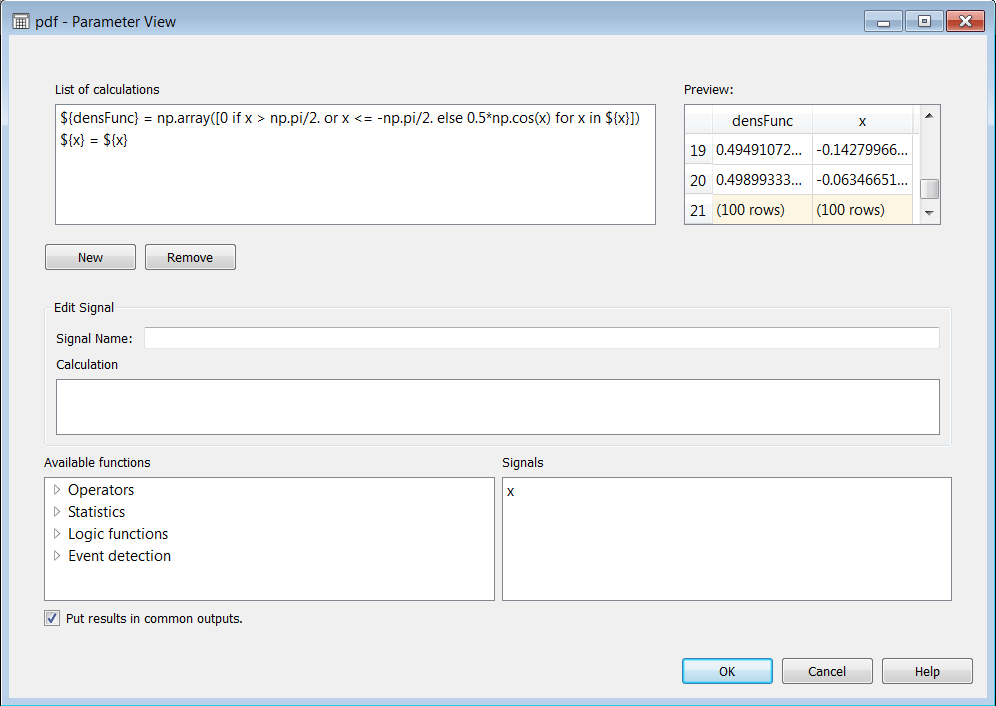We will use densFunc and x to evaluate how well our approximation behaves in the end.

Now, building a CDF from this easily integrable analytic function is quite simple. You can go the analytic or the numeric route. For this tutorial we will also show the numeric route because not all pdfs you may run into will have an easily integrable analytic form. We use the trapezoidal rule just to show that the PIT will approximate the original random variable X even with a not-so-smooth approximation of the CDF. To build the CDF, we take each value of the integration and sum it with the previous value, like so:

np.array( [ ( (${x}[index]-${x}[index-1]) * (${densFunc}[index]+${densFunc}[index-1])/2.) + np.sum([ ( (${x}[indexK]-${x}[indexK-1]) * (${densFunc}[indexK]+${densFunc}[indexK-1])/2.) if indexK>0 else 0 for indexK in range(index) ]) if index>0 else 0 for index in range(0,len(${x})) ]) To analytically build a the CDF, you can start by integrating 1/2 cos(x) dx. Which will give you 1/2 sin(x) + C. To find C, solve the initial condition sin(pi/2) = 1, and that gives you 1/2 sin(x) + 1/2 as the function from which you can generate a CDF. Now, if we plot the PDF and the CDF together (you can use the plot tables node, and choose x as the independent axis), they will look like this: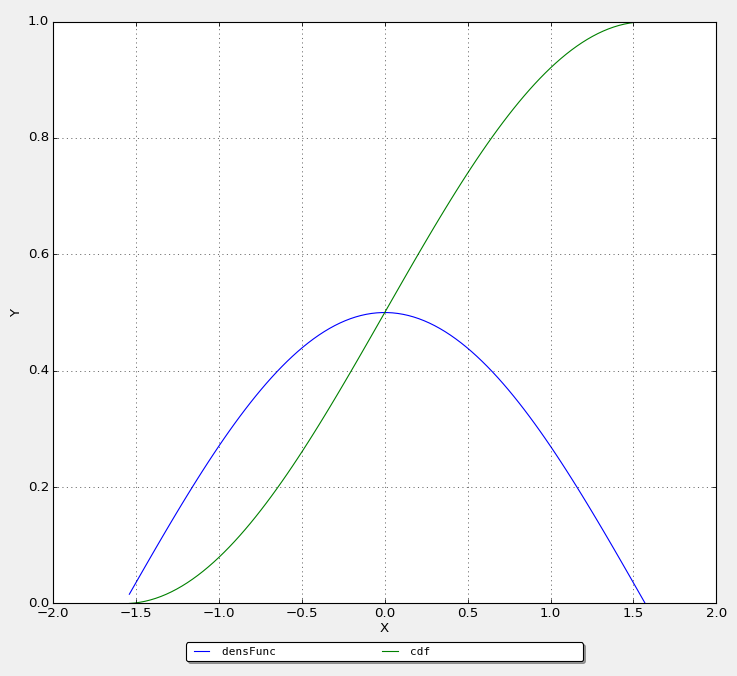Ok, NOW we have all we need to generate some data with the same distribution as X. And we will both use the numeric and the analytic ways. The general way to obtain a function of the uniform distribution is in slide 10 of the presentation, so solving for x: u = 1/2 sin(x) + 1/2 -> x= arcsin(2u-1) You can add a new calculator node, and feed the output of CDF to it. Let us take as many samples as elements exist in our initial independent axis (just for being able to use the same axis across different graphics), and name that simRandVarFromU. You can enter in the calculation field: np.array([np.arcsin(-1+2.*np.random.uniform(0,1)) for b in${x}])

Now, your friend in Carcass Island may actually find it easier to use the numeric way. He probably has a lot of stones and writings on the sand (drawing a lot of slots for his coin to emulate different positions between 0 and 1), and he has been spending a lot of energy scaring away the sheep and the penguins, so let’s make his life a little bit easier. He can calculate numericSim by doing the following:

np.array([${x}[np.where(${cdf}<=np.random.uniform(0,1))[-1]] for inx in ${x}]) See what he did there? he rolled his coin until he got a uniformly distributed number ”u” between 0 and 1. Since he knows that the range of the CDF is between 0 and 1, it is safe to see in which value of x was the CDF less than or equal to ”u”, and take that as an index to obtain ”x”. After building the two estimates of X, let us find a way to compare them. So, what we are ideally constructing is a way of building a variable with a distribution as close as possible to X. So, let’s compare the distribution of: • Original X • PIT generated X (analytical) • PIT generated X (numeric) For both PIT – generated cases, we first obtained a histogram, applied a smoothing over the histogram, and then re-sampled the smooth histogram for a linear space like the one in the independent variable of Original X.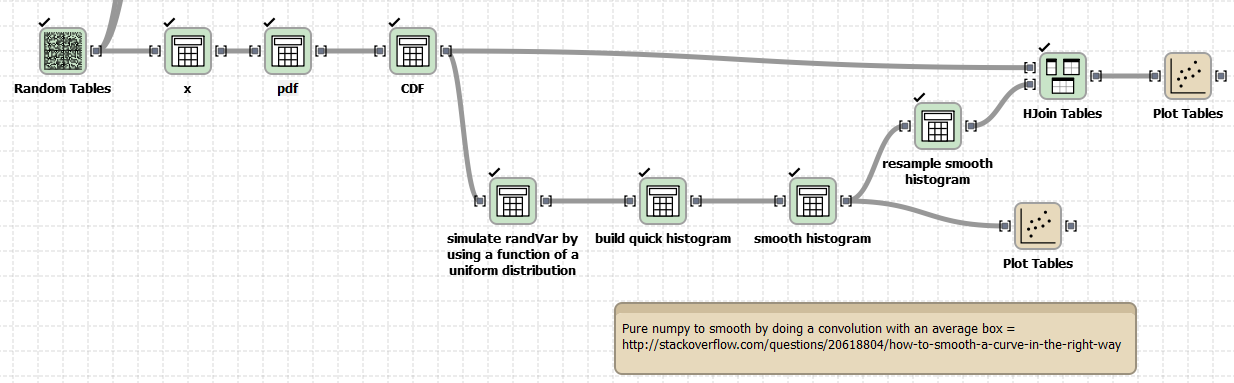To build the histograms: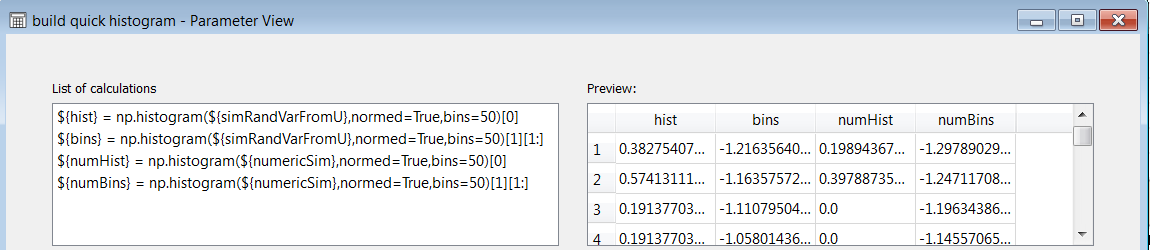Notice that we removed a bin since numpy will generate 1 extra bin. To smooth the histograms, we use a convolution with a window size of 10 samples, like this: np.convolve(${numHist},np.ones(10)/10,mode=’same’)

For both PIT generated histograms. Remember to add all the other signals to be able to make proper plots. So here is a plot of both smooth histograms and their bins: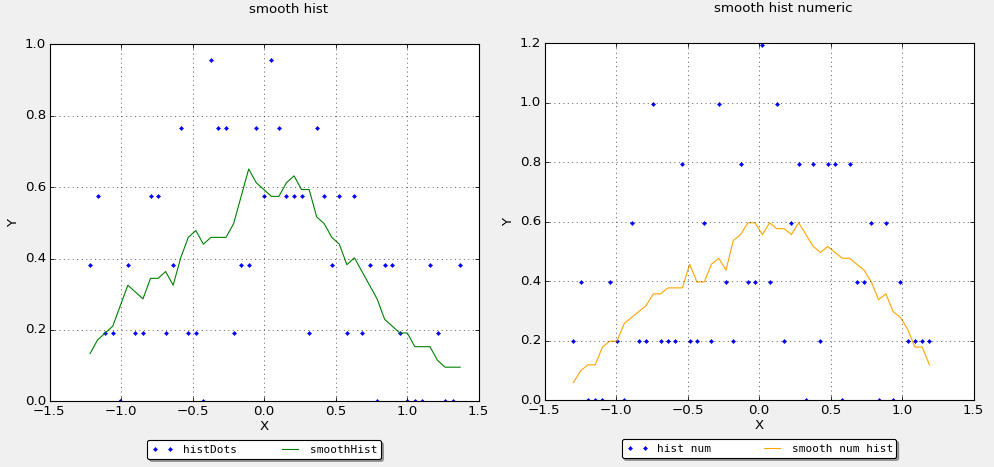To be able to compare the smooth histograms and the real PDF in the same plot, we made an interpolation (to change sample size). We simply did: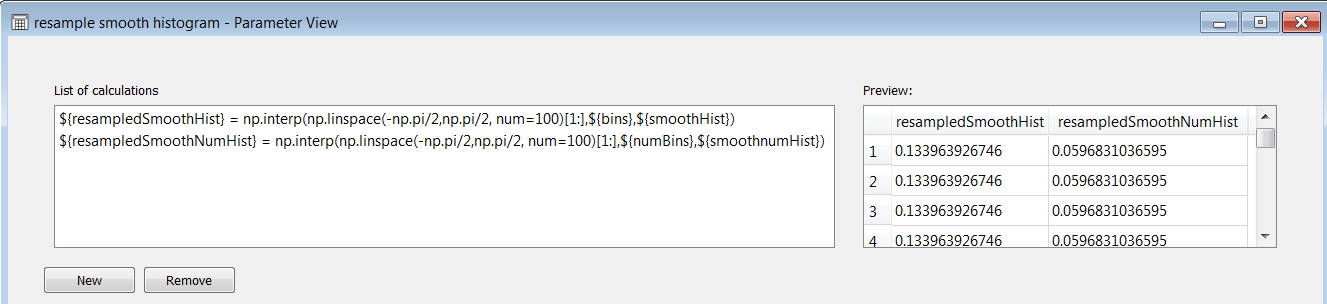And after putting together all signals on the same table, we were able to produce this plot: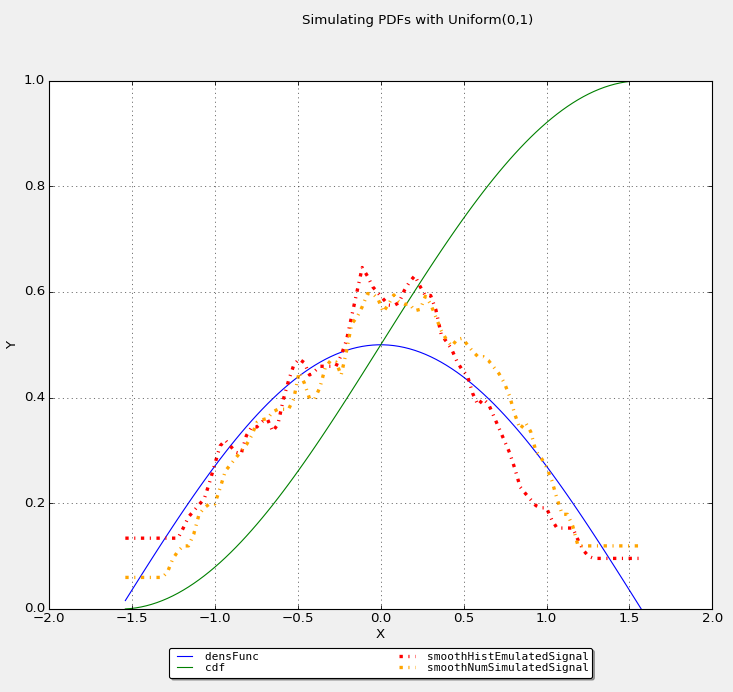So, one can see that at least with 100 samples and a smoothing window of 10 samples, with 50 bins, we got this approximation. What would happen if we start playing with this parameters? First, changing the number of bins at this small amount of samples would not make much difference. Second, getting more samples is very cheap for you in your desktop, but changing a convolution window would be cheaper for your friend in Carcass. An increase of a smoothing window to 23 samples will give us: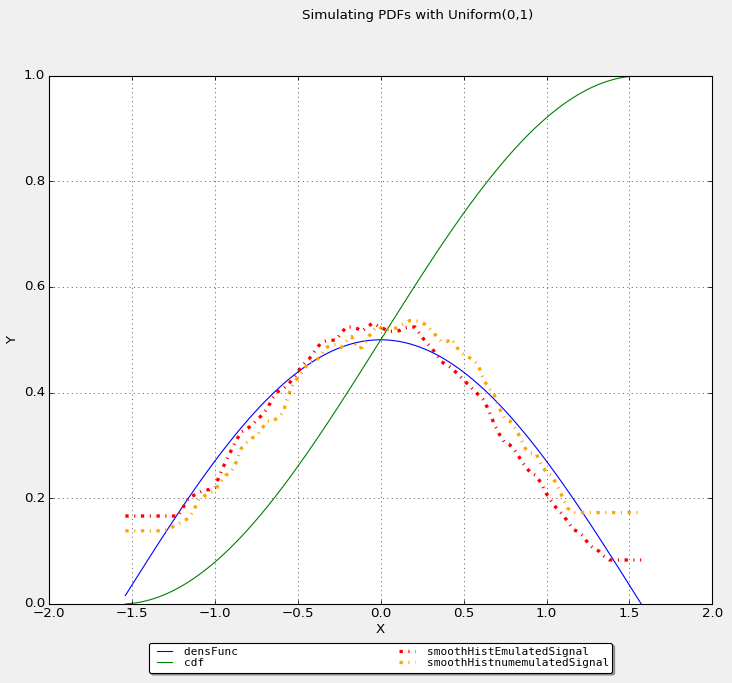But if we really wanted to see if the signals converge together, throw a bigger number of samples. Say, throw 1000 samples, and leave the smoothing window at size 10: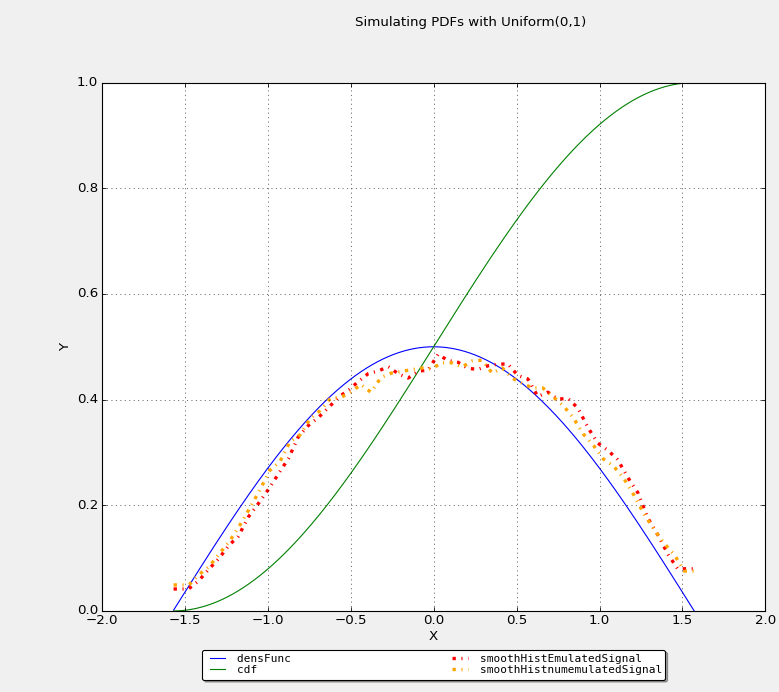I hope that you can add the PIT to your back of tricks, and use it whenever you have the need. Until the next post!

This post borrowed the featured image from here.

Or ”Martingales are awesome!”.

In a previous post, we talked about bounds for the deviation of a random variable from its expectation that built upon Martingales, useful for cases  in which the random variables cannot be modeled as sums of independent random variables (or in the case in which we do not know if they are independent or not, or even their distribution). We briefly explained that a martingale sequence is a sequence X_0, X_1,… so that for all i>0:

E[X_i | X_0,…,X_{i-1}] = X_{i-1}

and also:

E[X_i] = E[X_0] for all i≥0.

We discussed two bounds over variation of expectation when we have a Martingale (Azuma and Kolmogorov-Doob). However, the point of treating random variables as Martingales was not touched.

And, how exactly can I treat any random variable as a Martingale?

Easy, cowboy. Consider a probability space (Ω,F,Pr), in which Pr is a probability measure assigned to a field (Ω,F), from which Ω is a sample space, and F is a collection of subsets of the sample space Ω. One can see a sample space as an arbitrary set containing all possible outcomes in a probabilistic experiment. Events are subsets of the sample space. For instance, if the experiment is a sequence of two coin-flips, then Ω contains {hh,ht,th,tt}. An event ε ⊆ Ω for the experiment of two coin-flips can be the cases in which we get different values on each coin flip, containing {ht,th}.  The collection of events in F must satisfy:

1. ∅ ∈ F
2. ε ∈ F ⇒ complement of ε also ∈ F (closure under complement)
3. ε1, ε2,… ∈ F ⇒  ε1 ∪ ε2 ∪ … ∈ F (closure under countable union)

Closure under complement together with closure under countable union also imply closure under countable intersection. For a field in which F = 2^Ω, is possible to build sequences of nested subsets of F so that F_0 ⊆ F_1 ⊆ … ⊆ F_n. From that, we can see that F_0 contains the empty event, and F_n contains 2^Ω. Those sequences of nested subsets of F is what we call a filter or filtration in the context of probability spaces. Given an arbitrary F_i in a filter, we can say that an event ε ∈ F is F_i-measurable when ε ∈ F_i.  This basically means that an event can only be measurable in a filtration, if the subset of the sample space associated to that event is also contained in the filtration. As a consequence of filtration being nested subsets, if an event is F_i-measurable, then the event is also F_{i+1}, F_{i+2},… and definitely F_n measurable.

Now consider a random variable X over a probability space (Ω,F,Pr). X can be viewed as a function X: Ω → R, meaning that for a given sample ω ∈ Ω, the random variable will take the value X(ω) ∈ R. In other words, we have a random variable that is used to associate a random sample in the sample space with a number in the domain of the real numbers. Considering the F_i-measurability concept, we can say that the random variable X is F_i-measurable if for each x ∈ R, the event {X = x} is contained in F_i. That also means that X is F_j measurable for all j≥i.

Starting from this random variable X, we can build our Martingale. What can be said about X with respect to F_{i-1}? Since X is a function over the values of Ω, we know that the values X will take will be constants over each event. X is a function and its value does not have to be a constant for a sequence of events. It can have a different behavior. However, X is well defined enough to have an expected value over a sequence of events, and such expected value is:

E[ X | F_{i-1} ]

Which is the expected value of our random variable X over the events contained in F_{i-1}. And this expected value is in itself another random variable. A random variable that constitutes a mapping from F_{i-1} into the reals. An interesting property of this random variable, is that its value is constant if X is also F_{i-1}-measurable. This means that the expected value of X given F_{i-1} will be constant as long as there are events in F_{i-1} for all values X can take. And of course, there is the case in which E[ X | F_{i-1} ] can be constant if E[X] is constant, when X is independent of F_{i-1} (since then, from conditional expectation rules, E[ X | F_{i-1} ] will simply be E[X]).

Putting everything together: for a probability space (Ω,F,Pr) with a filtration F_0 ⊆ F_1 ⊆ …, and a sequence of random variables X_0, X_1, …, so that for all i≥0, X_i is F_i-measurable. Then, the sequence X_0,… X_n is a martingale, as long as for all i≥0,

E[ X_{i+1} | F_i ] = X_i

Any subsequence of a martingale is also a martingale, relative to the corresponding subsequence of the filter. Considering that, and knowing that X is a random variable over the probability space, then is possible to define:

X_i = E[ X | F_i ]

and it will follow that the sequence X_0,… X_n is also a martingale. This is how you construct a martingale from a random variable, and is also known as a Doob martingale. The key point is that each for each 0≤i≤n, X_i is F_{i-1}-measurable.

So, I can treat a random variable as a Martingale. What can I do with that?

You could do this. And while you are at it, you can consider the following:

• All martingale properties are applicable to this martingale sequence.
• The expectation variation bounds with martingales discussed in the previous post are applicable. Those are powerful bounds since they are not so restrictive.
• Constructions of martingale differences is possible by having Y_i = X_i – X_{i-1} and requiring E[Y_{i+1} | F_i] =0

But if you want some examples, we can give you some ideas to build a relaxation of your real application.

Polya’s Urn Scheme:

Consider an urn that initially contains b black balls and w white balls. We perform a sequene of random selections from this urn, where at each step the chosen ball is replaced with c balls of the same colour. Let X_i denote the fraction of black balls in the urn after the ith trial. Show that the sequence X_0, X_1… is a martingale.

To prove this, we will use a property of martigales, by which for all i≥0, E[X_i] = E[X_0], and we will first take the trivial case, and use it to build a general case.

First, let’s take the trivial case. The value of X_o is a constant depending on b and w, which is which is b/(b+w). Since the expected value of a constant is the constant, then E[X_o] = X_o .

Now, let’s build an expression for the general case of X_i. Since at each trial we replace the chosen ball with c balls of the same colour, then on every ith step we add in total (c-1) balls into the urn. This means that in any given point i, we will have in total:

b+w+i*(c-1)

balls. X is the fraction of black balls in a given time i, so that numerator becomes itself a random variable depending on the expected value of black balls at point i. Then, we can say that at a certain point i we will add (c-1) black balls with probability E[X_{i-1}]. We use E[X_{i-1}], since the fraction of balls in the urn (X) is a probability, but since the value of such probability is a random variable itself, then we talk expected values of that random variable. In this way, the numerator becomes:

b + expected value of black balls at time i

which is:

b + Σ (number of black balls added on each i) * (probability of adding a black ball at i)

which for all i>0 is:

b + Σ (from 0 to k=i-1) (c-1) * E[X_k]

Now putting numerator and denominator together:

E[Xi] = (b + Σ (from 0 to k=i-1) (c-1) * E[X_k]) /  (b+w+i*(c-1))

This expression holds for i>0. Let us first verify that this expression holds for i=1:

E[X_1] = (b + Σ (from 0 to k=0) (c-1) * E[X_0]) /  (b+w+(c-1))

E[X_1]= (b+ (c-1) * b/(b+w) ) / (b+w+c-1) = ((b*(b+w)+b*(c-1)) / (b+w)) / (b+w+c-1)

= (b*(b+w+c-1)) / ((b+w)*(b+w+c-1)) = b/(b+w)

So, E[X_1] = E[X_0]. For the nth case:

E[X_n] = b*(b+w+n*(c-1)) / (b+w)*(b+w+n(c-1)) = b/(b+w)

Again, E[X_n] = E[X_0]. Which is what we intended to demonstrate. Oh yeah. You can look at other examples for inspiration in 18.2.1 of here, the whole presentation here, and something a bit more hardcore yet well explained here.

The featured image of the title comes from here.

In the previous post  we looked at Chebyshev’s, Markov’s and Chernoff’s expressions for bounding (under certain conditions) the divergence of a random variable from its expectation. Particularly, we saw that the Chernoff bound was a tighter bound for the expectation, as long as your random variable was modeled as sum of independent Poisson trials. In this post, we will expand to the cases in which our random variables cannot be modeled as sums of independent random variables (or in the case in which we do not know if they are independent or not, or even their distribution). For that, this post will be on two inequalities involving Martingales: Kolmogorov-Doob and Azuma.

Martingales have attracted more than one, especially those related to gambling (for some anecdotal cases, check here). The martingales we refer to in this post, are sequences of random variables. So, for a sequence X_0, X_1,… if for all i>0:

E[X_i | X_0,…,X_{i-1}] = X_{i-1}

And this is known as a martingale sequence. Consequently, in martingale sequences, E[X_i] = E[X_0] for all i≥0. Taking of X_i as the capital of a gambler playing a fair game after the ith bet. In a fair game, both players have the same chance of winning, so the expected gain or loss from each bet is zero. So even if sometimes you win and sometimes you lose, gains and losses should cancel each other and converge at the initial value. Hence the phrase ”quit while you are ahead”.

When we get a sequence with the following property:

E[X_i |X_0,…, X_{i-1}] ≥ X_{i-1}

then the expected value of our capital would either remain or grow with each bet. It doesn’t mean we will never lose, it only means that time is in our side. And such sequences are known (unsurprisingly) as super-martingales.

The concept becomes even more appealing to gamblers when we define martingales in terms of net gains or losses from  the ith bet. So, being Y_i=X_i-X_{i-1} (or the difference between the capital of the gambler in bet i and in bet i-1) and X_i = X_0 + ∑Yj (from j=1 until j=i), we can get something called a sequence of differences (also named martingale differences). In martingale differences, it holds that for all i≥1:

E[Y_i | Y_1,…,Y_{i-1}] = 0

There is a way to construct martingale sequences from any random variable (also known as Doob martingales). It is also possible to convert super-martingales into martingales. But maybe that is a matter to talk about in another post. Maybe titled ”Martingales are awesome”.

Now, lets get into the matter of our expectation bounds. Now, how would a person deciding where to invest its capital feel if he/she could have an idea of when to ”pull out” of the investment (a.k.a the time i of expected maximum capital during the cycle). Or if he/she has provisioned enough to survive the roughest time of the investment?.

Kolmogorov-Doob bound:

Let X_0, X_1,… be a martingale sequence. Then for any λ > 0 :

Pr[max_{0≤i≤n} X_i ≥ λ] ≤ E[|X_n|] / λ

This form of Kolmogorov-Doob tells us that for a capital X, the probability of having maximum capital ≥ λ in any bet i of the whole game (a.k.a. that moment in which you are on fire) is bounded by E[|X_n|]/λ.

Notice that E[|X_n|] is the sum of the product of all possible positive AND negative values times their probabilities (shamelessly citing wikipedia here). Following that, if there was an equal probability of X_n taking any value between X_n and -X_n, then E[|X_n|] would become 0 and supporting myself on |E[X]| ≤ E[|X|], and being |E[X]| non zero if X0 is nonzero, then it must apply that in a martingale, the probabilities of having any specific value of X_n between X_n and -X_n are not the same for every value, unless (possibly) X_0 was 0 -interestingly, I have not found explicit restrictions over the value of X_0-. If you wanted to be pessimistic and lazy, you could use |E[X_n]|/ λ = |X_0|/λ as your bound instead -if the worst case scenario does not look so bad and you have no other information, you may as well dwell with care into it-. You may optionally scream ”YOLO” for courage.

Azuma’s bound:

Can be found in other textbooks as Hoeffdings’s inequality. Let X_0, X_1,… be a martingale sequence so that for each for each k:

|X_k – X_{k-1}| ≤ c_k

where c is independent of k. Then, for all t≥0 and any λ >0,

Pr[|X_t-X_0| ≥ λ] ≤ 2e^( (-λ^2) /(2*∑(c_k)^2 (for k=1 to k=t) ) )

This bound over martingales resembles the exponential bounds in Chernoff’s inequality. It basically tells us that the probability of X deviating more than λ from its initial value in a bet t, is bounded by an exponential function, given that the successive differences in each bet are less than or equal to a certain quantity c. The process of applying this bound to a martingale sequence is also known as the method of bounded differences.

To apply any of the former methods you would have to of course do your homework and find out as much information as you can on the system from which the martingale is generated. Sometimes it can be useful to build the martingale from a relaxation of the system, make your calculations over it, and see if your relaxation is harder than how the system is more likely to behave. And there are many other useful bounds over martingales that are not in this post. In other words, if you can possibly model a system’s behaviour with martingales, you can get a much better idea of the system without necessarily relying on experimental data (see Polya’s urn problem in our next post, ”Martingales are Awesome”).

Most information in this post was taken from section 4 of the Randomized Algorithms book from R. Motwani and P. Raghavan, and from sections 7 and 12 from the Probability and Random Processes book from Grimmett and Stirzaker.

In our previous post, we briefly explored the plotting capabilities built in Sympathy, and also the enormous flexibility that the calculator node brings into our flow. Today we will use those two nodes to build and plot one of the simplest, most widely used models of data: a linear regression.

Our flow will simply have four parts:

1. A data capturing/manipulation part (that we use to obtain/import/filter the measurements and sample number / time information),
2. A part for obtaining the model coefficients
3. A part for generating a plot of the model together with the input data
4. A part for exporting the data and plots.

In the following figure, parts 2-4 are visible.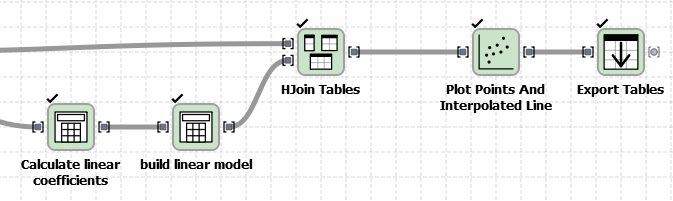For some help on how to do part 1, you can read here.

Regarding part 2, we will use the calculator node. More specifically in the flow in here, is the node in the leftmost bottom part that says ”Calculate linear coefficients”. Our configuration screen looks as follows: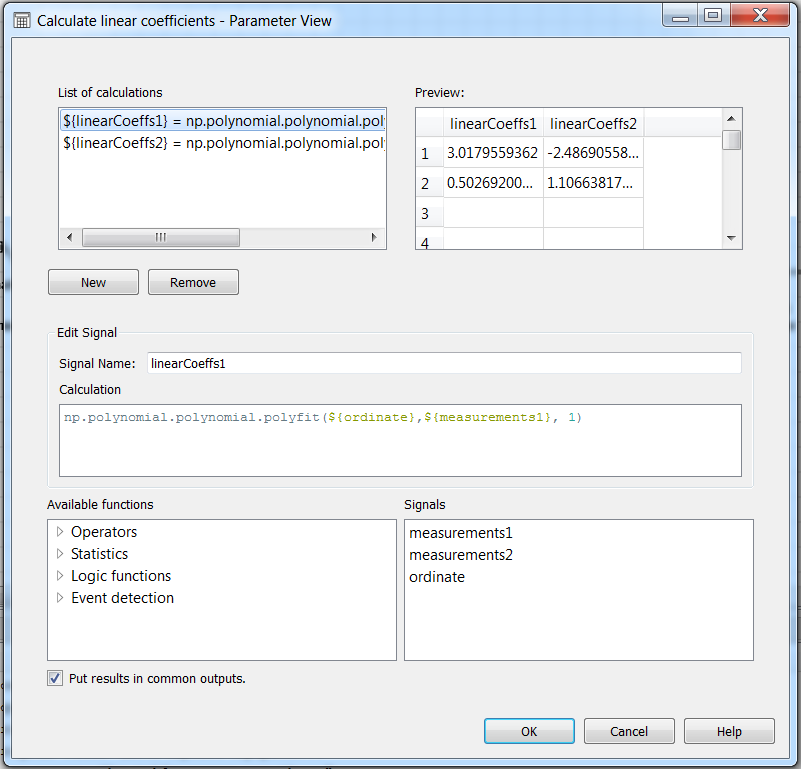The two input signals have the same size and were generated with the same time axis. As you can see, we are using polyfit in numpy for obtaining the model coefficients in each signal (see numpy documentation). This means that if you know your data can be better fit to another polynomial, you could request a different degree for the coefficients. In this example, for the two measurements, we have requested a first degree. Measurement 1 is more or less well behaved, while Measurement 2 is much noisier.

Now, the output of this calculation operation will be the two coefficients, ordered by the degree of the coefficient, i.e. if the model is mX^1 + bX^0, then we will have that linearCoeffs1 = b and linearCoeffs1 = m.

With this in mind, we can go to the configuration of the next calculator node (the one that says build linear model) in order to generate the data for plotting the model: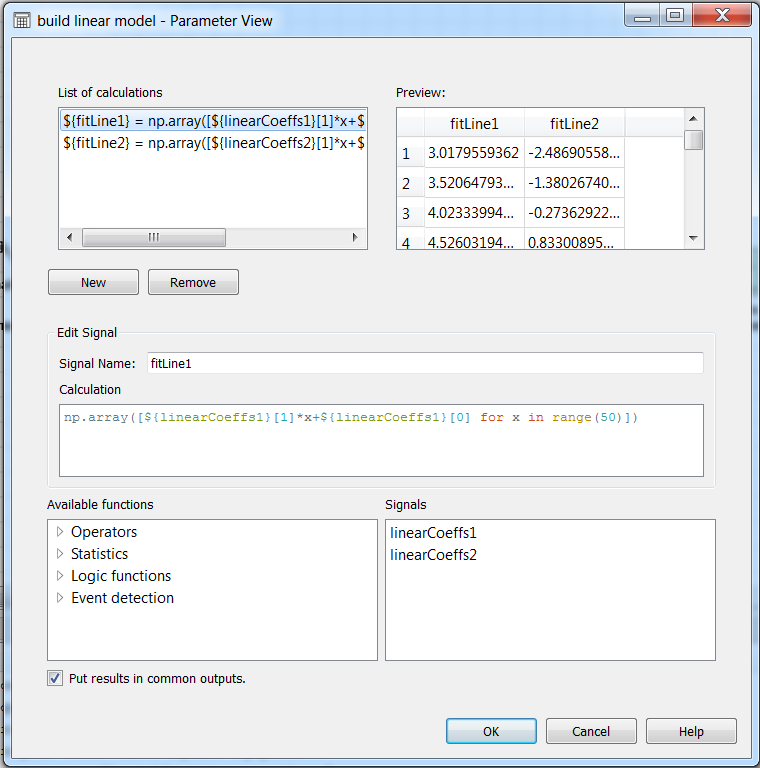So, since our linear model is Y = mX+b, then we generate a numpy array taking m and b from the linearCoeffs that we would like to plot, and we do that over the length of the original measurements (in this example it was 50 samples). In this way, the output signal from the plottable model is as long as the input data, and we can easily apply ”Hjoin Tables”, in order to have all the information we need to plot it.

After joining the original data with the models, we can go to the configuration of the plot node, and put together the model and the input data in the same canvas. You can play with the colors, the types of lines and markers, add a grid, place proper names, and even add the mean of each signal in the label (as the featured image in this post).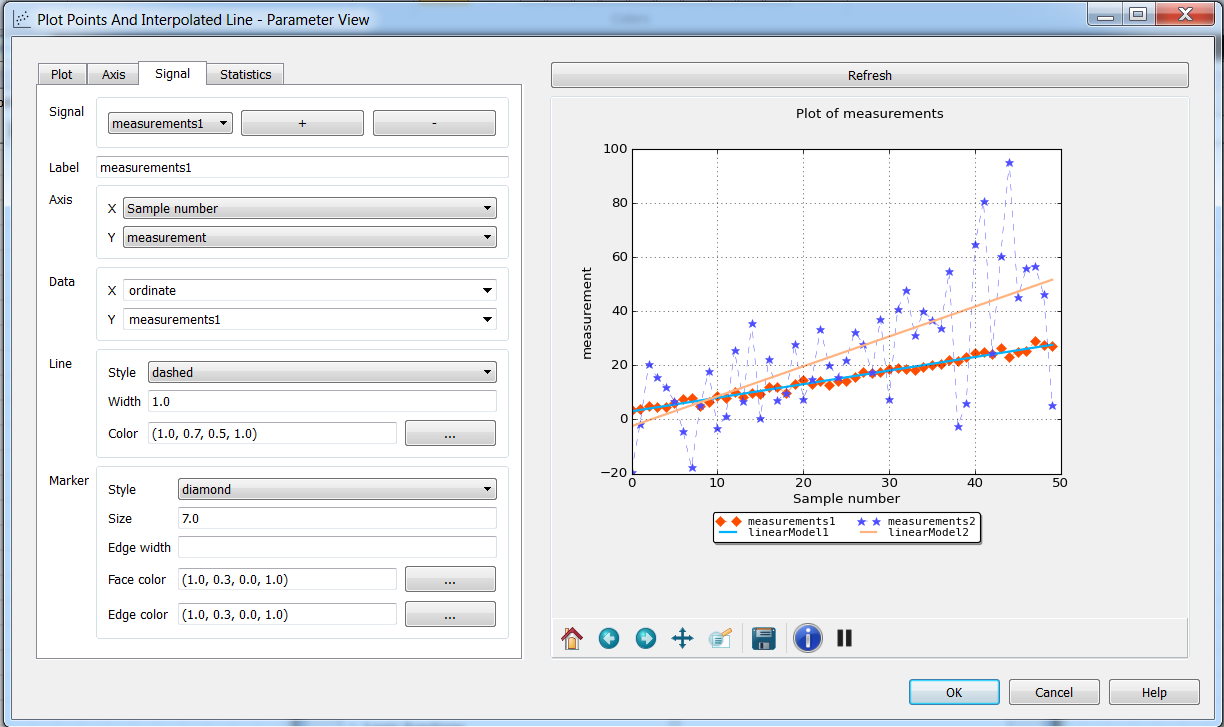The final step is exporting. A common use of the plotter in sympathy is as an inspection tool for signals that we have in our data, in that way we may notice if we need to filter the data a bit better or if the signals are behaving as expected. Then, to export the plots in the plotting node, we feed  the signal into an exporter, and we choose the type of file we want to export the graphic in ”plot output extension”. We can choose between png, pdf, and a couple more. This will export both the data and the plot.

And you are set! I hope you have some nice holidays. I will do, for sure!

In a previous post we were discussing the pros and cons of parametric and non-parametric models, and how they can complement each other. In this post, we will add a little more into the story. More specifically, we are going to talk about bounds to the probability that a random variable deviates from its expectation. In these two posts we will talk about the following well-known techniques:

1. Chebyshev’s Inequality
2. Markov’s Inequality
3. Chernoff’s Bounds

They exploit the knowledge on some feature of the random variable e.g. the variance of the distribution, or the random variable being obtained from a poisson process, or knowing that the random variable does not have negative values. You can find extensions for other properties of the random variable under ”tail inequalities” or ”deviation inequalities”. Techniques 1 and 2 were developed in the last part of the 1800’s. The latest is a bit more modern (the author is still alive!).

Why would you want to estimate bounds over the probability that a random variable deviates from its expectation? Some applications rely on expected values to make estimates/predictions.  And on many cases, this is a reasonable assumption to make. If you want to use expected values to represent a random variable, or as part of another technique to provide an output in a decision making process, then is sensible to provide some bounds on the probability that the random variable may deviate from the expectation.

If you are lucky enough to find a problem (or a simplification of a problem) in so that it satisfies all conditions necessary for all techniques, the order of ”tightness” of the bounds is Chernoff-Chebyshev-Markov, being Chernoff the tightest. This is possibly the reason why, in spite of Chebyshev’s inequality being older, many textbooks choose to talk about Markov´s inequality first. It is not rare to see authors use Markov’s inequality in the proof for Chebyshev’s. Actually, is not rare at all to see Markov’s inequality while going thru proofs, simply because it requires so little from your random variable, so it became a staple in the pantry of statisticians.

Chebyshev’s Inequality

Take-home message: ”The probability that a random variable is at least t standard deviations away from its expectation is at most 1/t^2”

Condition: We know the variance of the distribution.

Boring footnote: Some notes use interchangeably the distance from the expectation and the distance from the mean, which for a big enough number of samples becomes reasonable. I chose to use the expectation instead because the demostration of Chebyshev via Markov uses a random variable composed of the absolute value of the difference between your random variable and its expectation, so is easy to remember. But both are probably just as fine.

Markov’s Inequality

Take-home message: ”The probability that a random variable takes values bigger than or equal to t times its expectation, is less than or equal to 1/t”.

Condition: The random variable must not take negative values.

Boring footnote:  It would make more pedagogical sense to start with Markov’s inequality, but I feel I need to start making some historical justice. If I find something older and just as useful as Chebyshev’s, I will probably make a newer post and scorn myself. Like this.

Ok, sorry about that link. Here, have some eyebleach.

Chernoff’s Bounds

Take-home messages:

”The probability that a random variable X built from the sum of independent Poisson Trials deviates to less than (1-δ) times their expectation μ, is less than exp(-μ δ ^2 / 2)”

”For the same random variable X, the probability of X deviating to more than (1-δ) times μ, is less than (exp(δ) / (1+δ)^(1+δ) ) ^ μ.

Conditions: The random variable must be a sum of independent Poisson trials.

This post will hopefully motivate you to study this topic on your own. If you do, some books you can check out:

The beautiful image for the preview is from here.

This post is my interpretation of Chapter 10 of the book ”Advanced Data Analysis from an Elementary point of view”. It is one of the most interesting reads I have found in quite some time (together with this).

Actually, the original title for the post was ”Book Chapter review: Using non-parametric models to test parametric model mis-specifications”. But shorter titles tends to attract more viewers.

The first question that one might ask is ”If we are going to use a non-parametric model to test a parametric model, why not going straight to non-parametric modelling instead?”.  Well, there are advantages to having a parametric model if you can build one. It could be of interest for your application to express your process in terms of known mathematical models. Also, a well specified parametric model can converge faster to the true regression function than a non-parametric model. Finally, if you only have a small number of samples, you could have better predictions by using a parametric model (even if slightly mis-specified). The reason is simply because parametric models tend to have a significantly smaller bias than non-parametric models. Also, for the same number of samples, a well specified parametric model is likely to have less variance in its predictions than a non-parametric model. Now, if you have a cheap and fast way to obtain many more samples, a non-parametric model can make better predictions than a mis-specified parametric model.

This is a consequence of the bias-variance trade-off that the author explains in a way that a person without a background in statistics can understand (in chapter 1.1.4 of the same book).

Non-parametric models ”have an open mind” when building a model, while parametric models ”follow a hunch”, if you will. One can find some similarities between modelling (parametric, non-parametric) and search algorithms, more specifically in uninformed search (BFS, DFS, Iterative deepening and variants) vs informed search (say, A* and variants). Even with a relaxed (and admissible) version of the optimal heuristic, A* is expected to traverse a shorter path than any uninformed search algorithm. However, it will traverse a larger path if the heuristic is poorly constructed, and most likely be outperformed by uninformed search. With this in mind, another question that may tickle you is: Can one translate a modelling problem into a search problem and have a machine automatically and optimally find the best parametric model for a given problem? Oh, yes (you can leave that as background music for the day if you like). You will of course need to express the problem in a way that the program terminates before our sun dies, and an admissible heuristic also helps. But yeah, you can do that. Humans often solve harder problems than they give themselves credit for.

If you were to build such a machine, the author can give you some suggestions to check for errors in your model. For instance, he suggests that if you have a good reason to think that the errors in a model can ONLY come from certain mis-specifications (say, that instead of being Y= θ1 X + ε it can only be Y=θ1 X + θ2 X + ε or maybe a couple other forms) then it may probably be faster and less sample-hungry for you to simply check whether the estimated θ2 is significantly different from 0, or whether the residuals from the second model are significantly smaller than those from the first. However, when no good reason is available to argue for one or other source of mis-specification, you can use non-parametric regression to check for all sources by doing either one of the following:

• If the parametric model is right, it should predict as well as, or even better than the non-parametric one. So, you can check if the difference between Mean Squared errors of the two estimators is small enough.
• If the parametric model is right, the non-parametric estimated regression curve should be very close to the parametric one. So, you can check that the distance between the two regression curves is approximately zero in all points.
•  If the parametric model is right, then its residuals should be patternless and independent of input features. So, you can apply non-parametric smoothing to the parametric residuals and see if their expectation is approximately zero everywhere.

For the last method, I can elaborate on the explanation from the author. If the residuals are Y-f(x;θ), then the expected value of the residuals given an input X is E[Y-f(x;θ)|X] (the author did not made it explicit, but I assume that the residuals must be calculated with x ⊆ X. I could be wrong on this, so please correct me if I am). Now, being our typical regression model something in the lines of Y=f(X;θ)+ε, we substitute this in the expectation, and we end up with E[f(x;θ)+ε-f(x;θ) | X]. In this expression, we have that f(x;θ)+ε-f(x;θ) = ε, so you end up with E[ε|X]. Since the constant is independent from X, then the expression becomes E[ε|X] = E[ε]. Since the expected value of a constant ε will always be the constant, then E[ε]=ε. And with a significantly small enough ε, we can say that ε ≅ 0, so no matter what input X we have, the expected value of the residuals of the predictor should be approximately equal to zero.

So yes, under some circumstances (too little samples) you can actually be better off with a slightly mis-specified (i.e. relaxed) model, than with a full non-parametric model. And yes, you can indeed check if the assumptions for your model are actually valid.

Have fun, and see you in the next post!

Allow me to introduce you to your new best friend from Sympathy 1.2.x: The improved calculator node. The node takes a list of tables, from which you can establish a new signal with the output for a calculation. There is already a menu with the most popular calculations and a list of signals from the input tables, all in the same configuration window.

To do this tutorial, it is recommended to use some data in csv format, and the latest version of Sympathy for Data (1.2.5 at the time of writing this post).If you would like to more complex formats (say, INCA-files) here are some pointers that can help you on that.

Now, with your data set and ready, follow the steps in here and locate the nodes to build the following flow:

And then right-click on the ”Calculator” node to open the configuration window for the node. Now, lets get our first calculation going!

Our First Calculation: A Column operation

1. Write a Signal Name (you don’t have to do it as the first step, but it helps you keep track of your work). In this example, we have chosen to write ”sumA” since we intend to obtain the sum of the values of a signal whose name starts with A.
2. Drag-and-drop a function from ”Available functions” into ”Calculation”. In this example, we have chosen to drag ”Sum”.
3. Drag-and-drop a signal name into approximately the point in the calculation in which the variable name is supposed to go.

You may need to delete some remaining text in the calculation until the preview in the top right shows you a correct number. It should look similar to the following screen: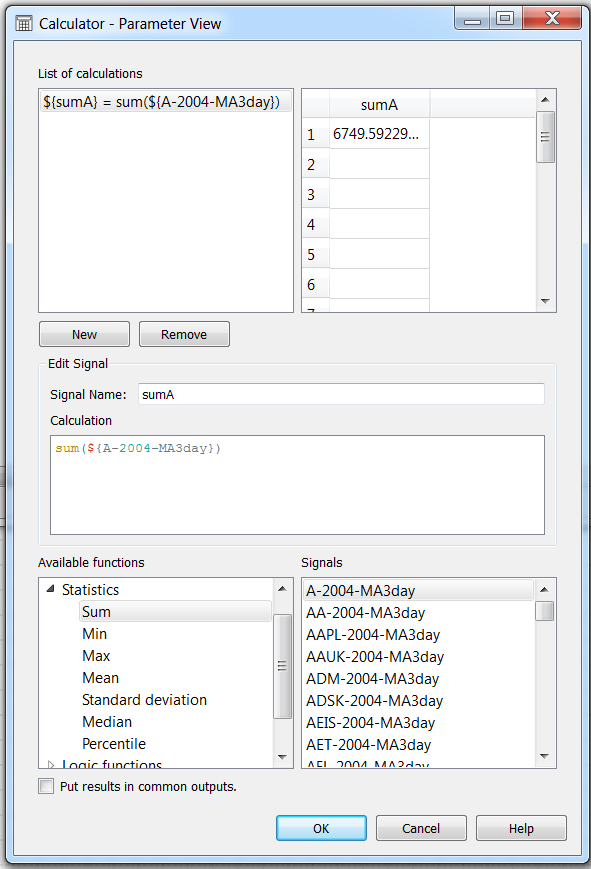First calculation. Note that the preview in the top right shows you a valid value

Second Calculation: A Row-wise operation

Now lets create a new calculation: click on ”New” (on top of ”Edit Signal”). We will now make a new signal that will be the sum of two signals, elementwise. As you can see in the figure below, this signal will be called ”sumTwoSignals”. So, simply drag-and-drop the names of the signals you want to sum, and make sure you put the operator between the two names. In this case, the operator was a sum (+).

What happens if the calculation outputs differ in shape?

In many cases (like in this example), the calculations may not have the same dimensions, and you may want to separate them into different output tables, because then Sympathy will inform you that it can not put together two columns with different lengths on the same table. For those cases, uncheck the ”Put results in common outputs” box, and then the results will go to different columns. In newer versions of sympathy the checkbox ”Put results in common output” is checked by default.

Now, lets go into something more interesting…

You can use the principles of the previous two sections together with the operators in ”available functions” in order to accomplish many day-to-day tasks involving calculations over your data.

But there is more fun from where all of that came from!. The real hidden superpower of your new best friend relies on the fact that you can access numpy from it. Yes, you can have the best of both worlds: the power of numpy from a graphic interface! Your colleague can stay in French Guyana and watch some space launches, you have almost everything you can ever dream of!

For our next example, lets build a binomial distribution. All you need to do is to write in the calculation field:

np.random.binomial(number_of_tests,success_pr,size=(x))

In which ”x” is the number of instances for the trials. This will give you a signal of size ”x”, from which each element in the output signal is the number of successful outcomes out of ”number_of_tests” for an event with probability ”success_pr” of succeeding. You can now manually assign a value to those elements. Notice in the figure below that you can either hand-type a set of values in there for quick estimations, or use variable names from input signals as inputs to the function.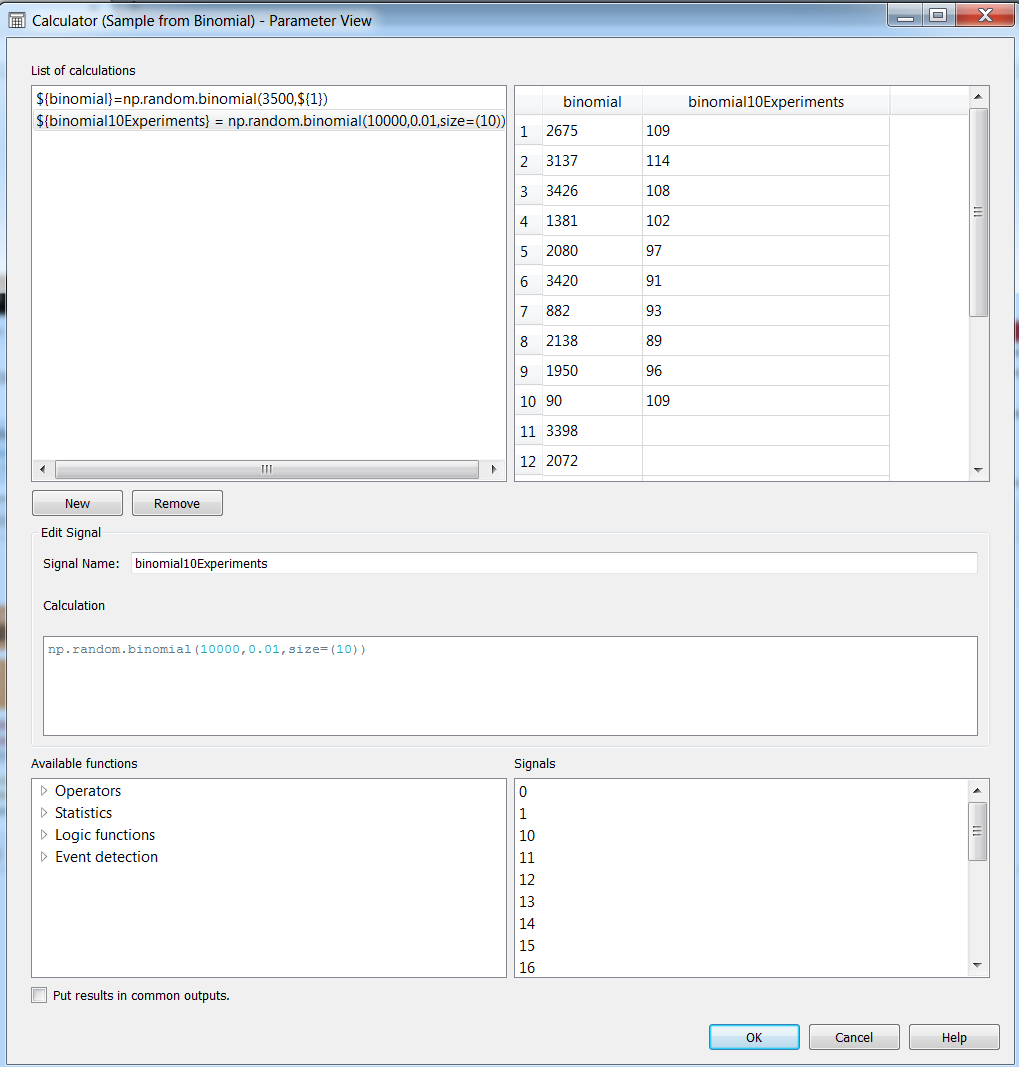Examples for using np.random.binomial with hand-written parameters and with signals from input files

Building and plotting a PDF (Probability Density Function)

A nice feature of the histograms in numpy, is that you can use them to build probability density functions by setting the parameter density to True (see numpy documentation entry here). Since np.histogram will return two arrays (one with the PDF, and one with the bin_edges) then you may want to also add a  in the end. So, it can look like this:

np.histogram(${yourVariable},bins=10,density=True) Now lets plot the distribution!. To be able to plot anything, since the plotting in sympathy is based upon matplotlib, you will require an independent axis, form which your PDF will be the dependent axis. You could take the other returning value of the histogram for that purpose, by writing a new calculation which will serve as the new independent axis for plotting: np.histogram(${NVDA-2006-MA3day},bins=10,density=False)[:-1]

Note that the length of the bin edges are always length(hist)+1, and here we have chosen the left as the start of the distribution.  A simple way to be clean about what you intend to plot, is to extract the signals and then hjoin then in a table, which will be fed to the ”Plot Table” module in sympathy. It can look like this:

In the configuration menu of the ”Plot Table” module, you can select the labels for the independent (X) and dependent (Y) axis, the scale, ticks and if you would like to add a grid into the plot. In the ”Signal” Tab, you can select which signal goes to which axis, and the colours and style of the plot. See below an example of a plot with 10 bins, a plot with 100 bins, and a plot with 1000 bins.

We hope that with this tutorial you can have some tools to study your data. And if you get bored and start daydreaming about your colleague at French Guyana, you can always watch this video. Have fun!

When your data is incomplete, somewhat corrupted or you simply need to use a black-box tool, you can help yourself by using statistics. Statistics build upon probability theory to draw an inference from our data.

Now, let’s get something out of the way: Not everyone has the same interpretation of the ”Probability” concept, and I will try my best to not to impose my view on yours. If you would like a nip on that discussion, check out this Wikipedia entry.

No matter what your view on it is, the quality of your inference depends mainly on the quality and quantity of data, the methods used for the inference and HOW the methods were chosen. How much do you really know about your data? Information on your domain can help you make assumptions to use techniques that do more with less data. While is entirely possible to infer something when you hardly know the domain, then you may need to use more ”data hungry” techniques. How much error/bias will you be introducing by using a technique when the assumptions do not entirely hold on the data? (and how much are you willing to accept for a clue?). You may hit a wall when you have a very small number of samples and little knowledge on the data. Then you should either get more samples, or get a better understanding on the data from other sources.

So here is what you can expect: Statistics are not magic. Getting good data is hard. Formatting data to make it useful requires work (which we hope tools like Sympathy can help you with). Choosing a set of techniques for your data is not a kitchen recipe, even though some sciences through the years have devised methods for specific situations. A method can get you information that is simply not there (bias), so be very careful and double check everything you find.

Econometrics theory is like an exquisitely balanced French recipe, spelling out precisely with how many turns to mix the sauce, how many carats of spice to add, and for how many milliseconds to bake the mixture at exactly 474 degrees of temperature. But when the statistical cook turns to raw materials, he finds that hearts of cactus fruit are unavailable, so he substitutes chunks of cantaloupe; where the recipe calls for vermicelli he uses shredded wheat; and he substitutes green garment dye for curry, ping-pong balls for turtle’s eggs and, for Chalifougnac vintage 1883, a can of turpentine.”

This quote from Cosma Rohilla Shalizi’s wonderful 800-page draft for his textbook ”Advanced Data Analysis from an Elementary Point of View”, in which he was quoting Stefan Valavanis, quoted in Roger Koenker, “Dictionary of Received Ideas of Statistics” s.v. “Econometrics”. When the circumstances surrounding your data are hard to control you may turn into the Swedish chef, but make do.

Now, lets get into the matter in the next post!